# 41+ Decimal Division Number Worksheet With Lesson Plan and Practice Guide

Decimal numbers are a system of numbers that has a decimal point to represent values less than one. A decimal point separates the whole number from the fractional part of the number. For example, the number 7.5 is a decimal number because it has 7 as the whole unit component and half or point five of another unit.

Decimal numbers are crucial measuring units and have different applications in money counting, measurements, and scientific calculations. It is one of the basic fundamental concepts in mathematics and one should have a strong understanding, especially for your young ones, while learning the initial mathematical lessons. They have a vast array of operations, such as addition, subtraction, multiplication, and division.

It is important to note that decimal numbers can be represented in different ways, including as fractions division or percentages. For example, the decimal number 0.75 can be written as the fraction 3/4 or the percentage 75%.

In this compilation, we have added 41+ Decimal Division Number Worksheet, which covers all the decimal number variations and use cases.

## 1. Decimal Division Worksheet Fifth Grades: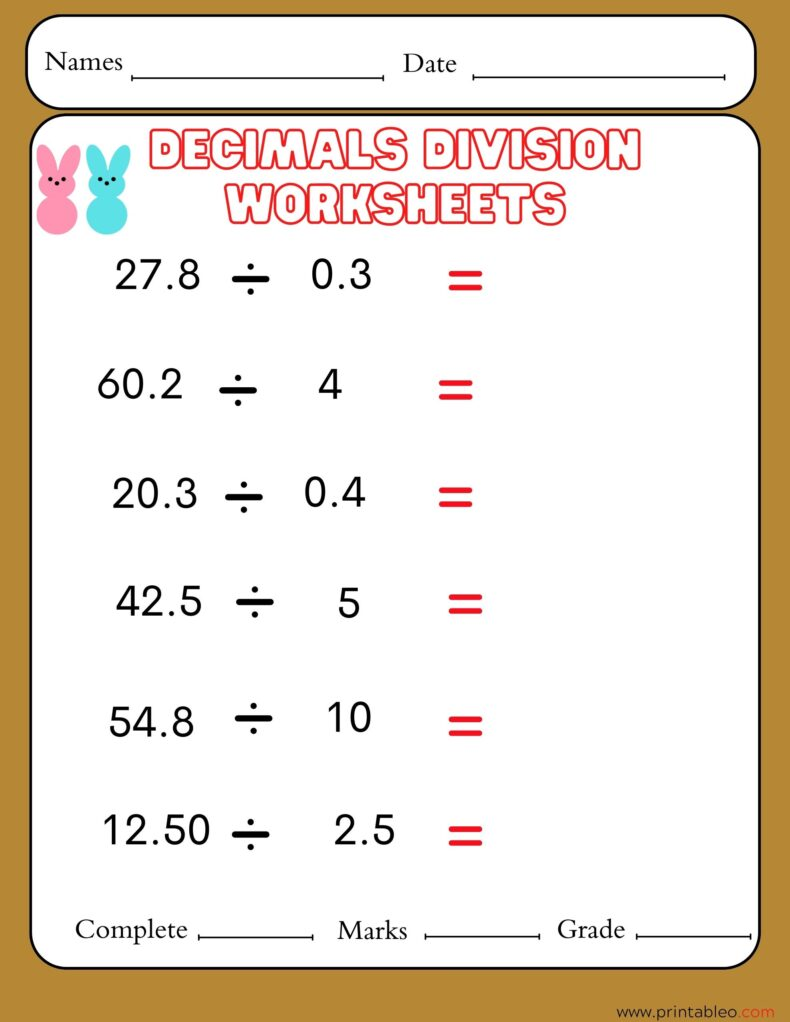## 2. Division By Decimals Worksheets Fourth Grade: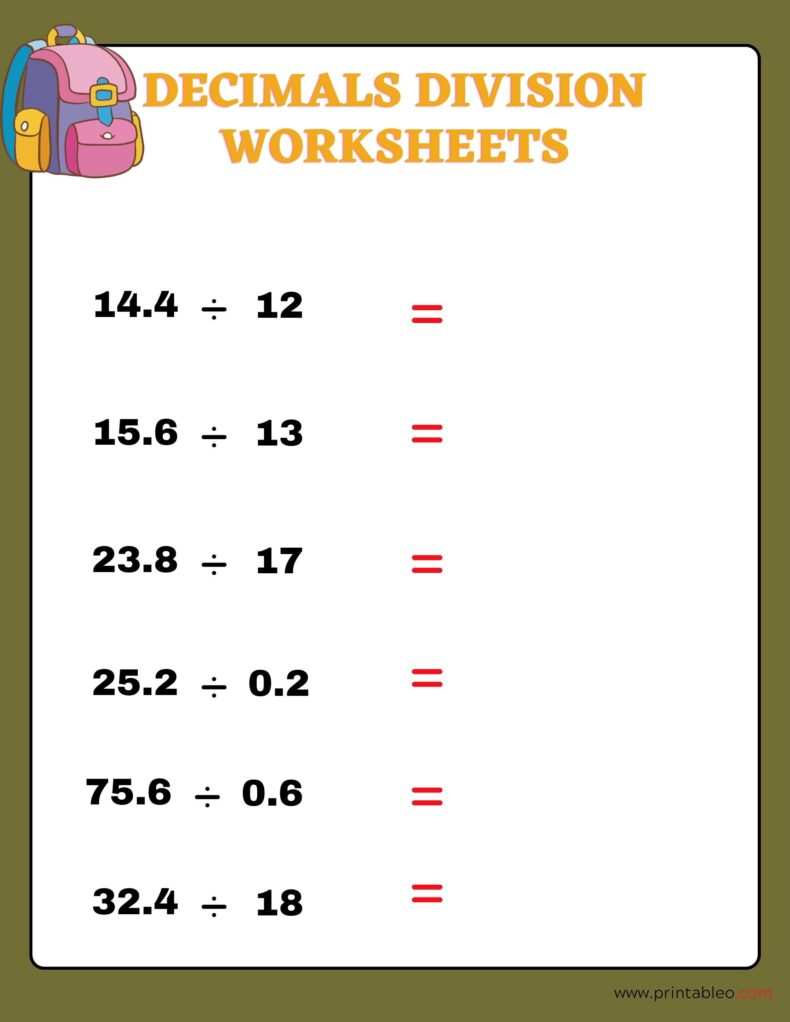## 3. Division of Decimals Worksheet 3rd Grade: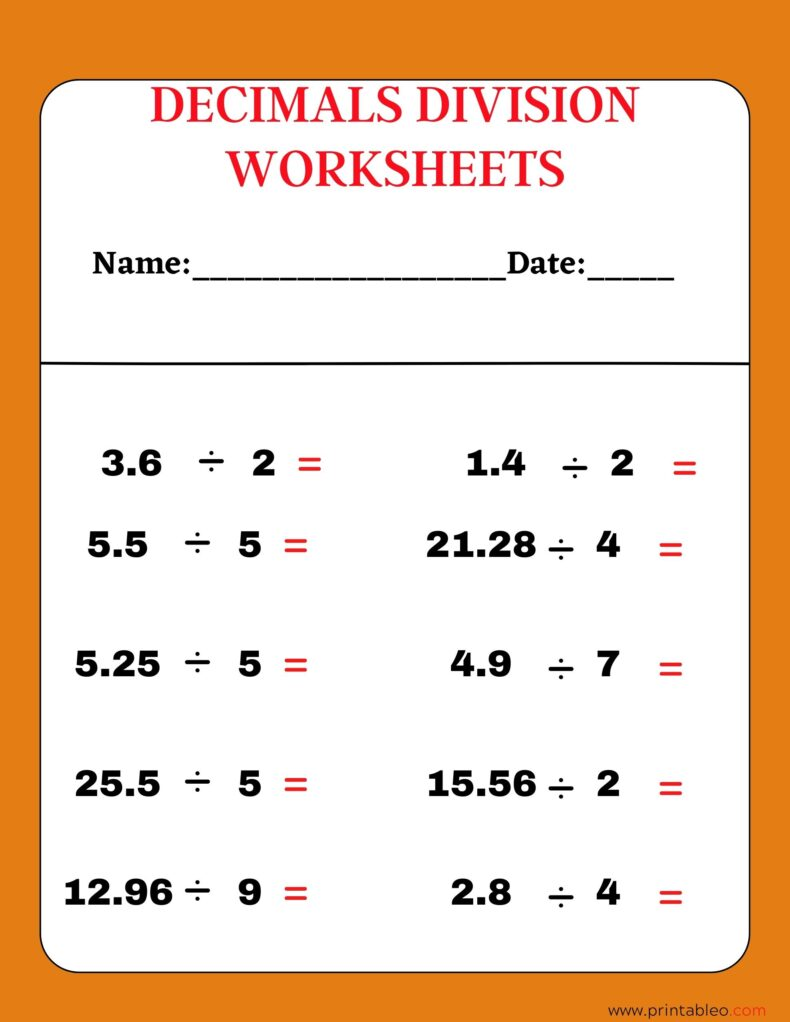## 4. Division By Decimals Worksheets: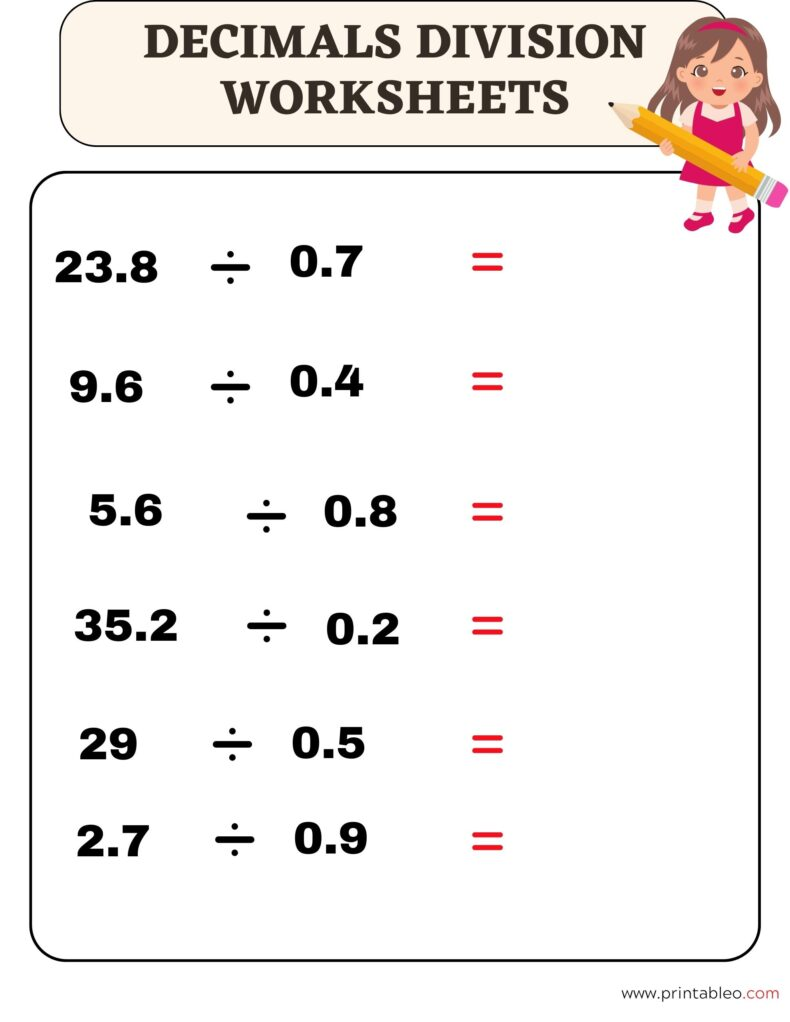## Decimal Division Worksheets and How to Use them for best possible results (Lesson Plan):

#### A) Overview of Our Worksheets:

Decimal division worksheets are effective practice sheets that work as tools designed to help students learn and practice so that they grow a fair understanding of dividing decimal numbers. All the listed worksheets contain a variety of problems, which covers dividing decimal numbers by other decimal numbers, whole numbers, or powers of 10.

We have made sure to present the worksheets in different formats, such as horizontal or vertical equations, word problems, or diagrams so that when practicing, students can face challenges from a different angle and grow a strong mathematical reasoning ability.

#### B. Example problems:

In the worksheets, we have presented Decimal Division Numbers from 3 different angles. Here are the use cases with examples:

1. Dividing a decimal by a whole number: Example: 6.2 ÷ 2 = ? Solution: Divide 6.2 by 2 to get 3.1
2. Dividing a decimal by a decimal: Example: 0.8 ÷ 0.2 = ? Solution: The Goal is to make the divisor becomes a whole number. Keep shifting the decimal point of both numbers to the right and then comes the normal division. In this case, 0.8 becomes 8 and 0.2 becomes 2. The equation becomes 8 ÷ 2 = 4.
3. Dividing a decimal by a power of 10: Example: 0.9 ÷ 100 = ? Solution: Follow the decimal division to the power of 10 rule.

D. Tips for solving problems efficiently:

1. First, start with the basic division with simple one-digit or two-digit whole numbers.
2. Try to increase speed and accuracy.
3. It is important for your young ones to double-check calculations to avoid mistakes that they do not form and wrong habits.
4. There is another important thing that might make or break is the decimal point placement. So pay close attention to avoid errors.
5. Kids can stats with scratch paper to keep track of calculations till they are comfortable and master the technics.
6. Do not start with longer complicated problems. Break them into smaller manageable parts.

If you want to improve your overall math skills and understanding, decimal division worksheets can be an effective tool. We have included 40+ diverse range of worksheets and by practicing with these, students can have a fair understanding of dividing decimal numbers and develop confidence and proficiency, which will eventually become a valuable skill in many different areas of math and daily life.

## 5. Division By Decimals Worksheets Third Grade: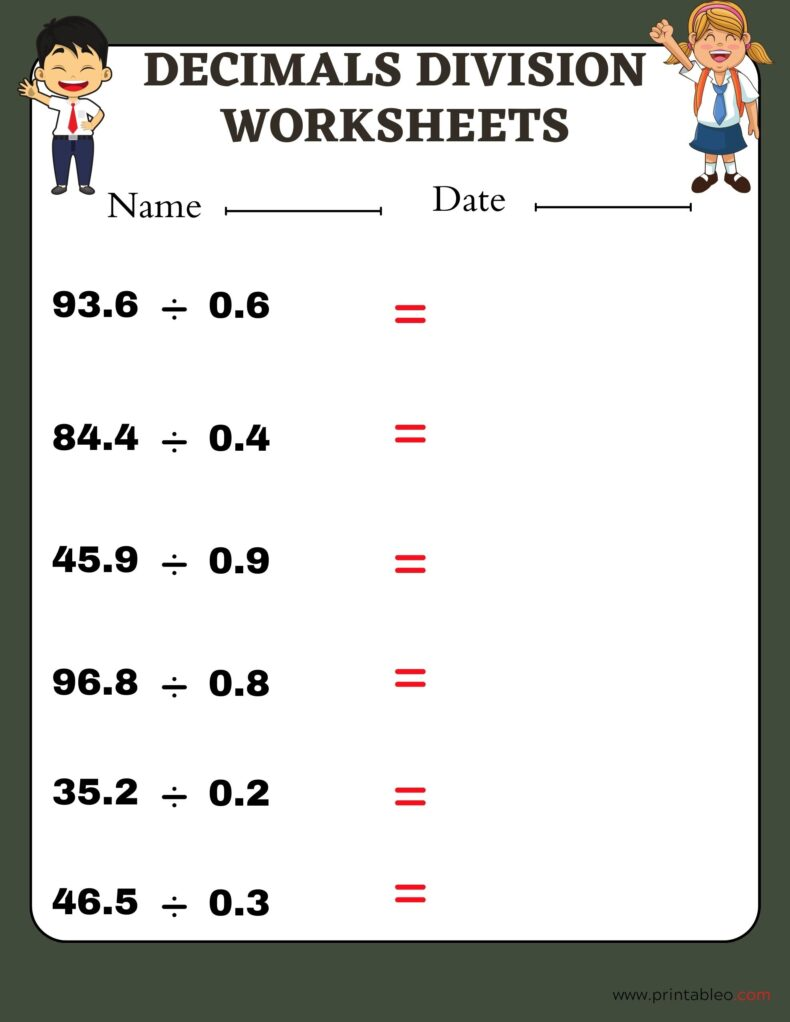## 6. Division By Decimals Worksheets Sixth Grade: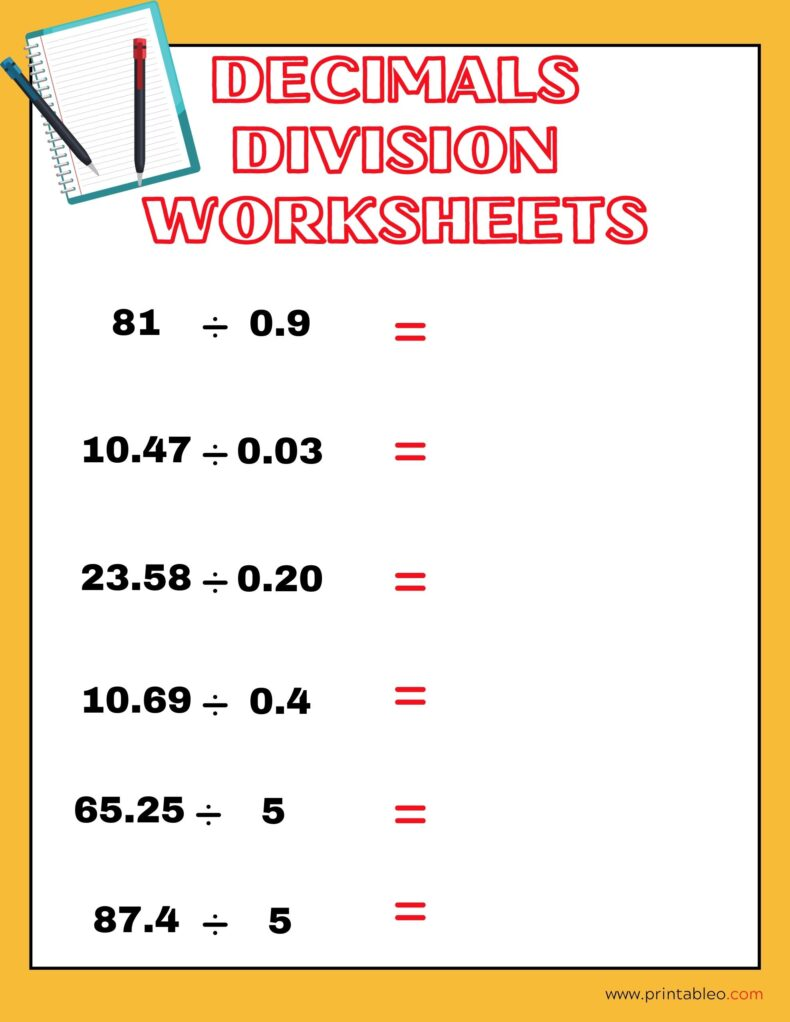## 7. Division By Decimals Worksheets Fifth Grade: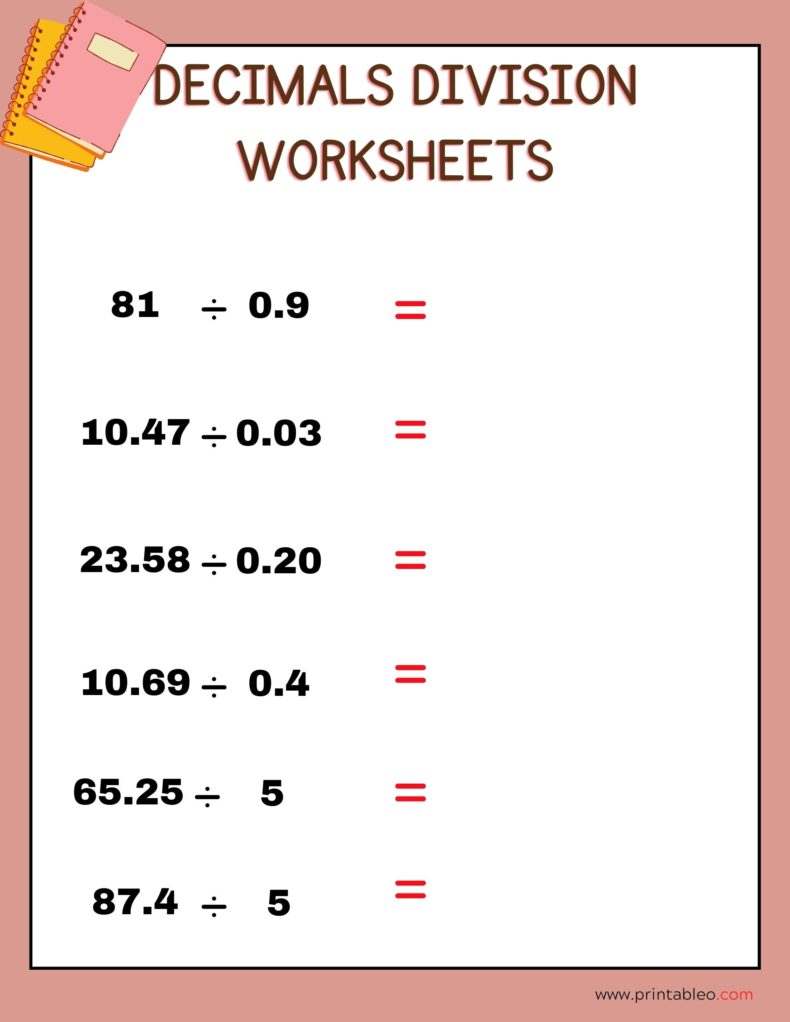## 8. Decimal Division Number Worksheet: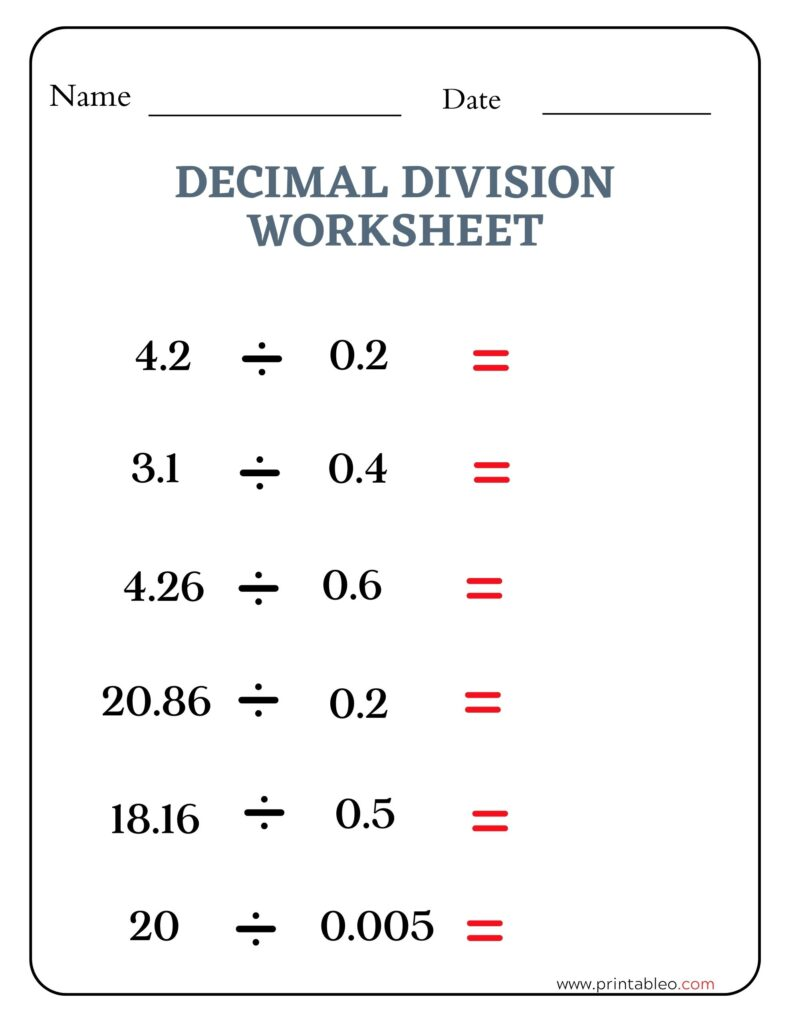## 9. Decimal Division Worksheet: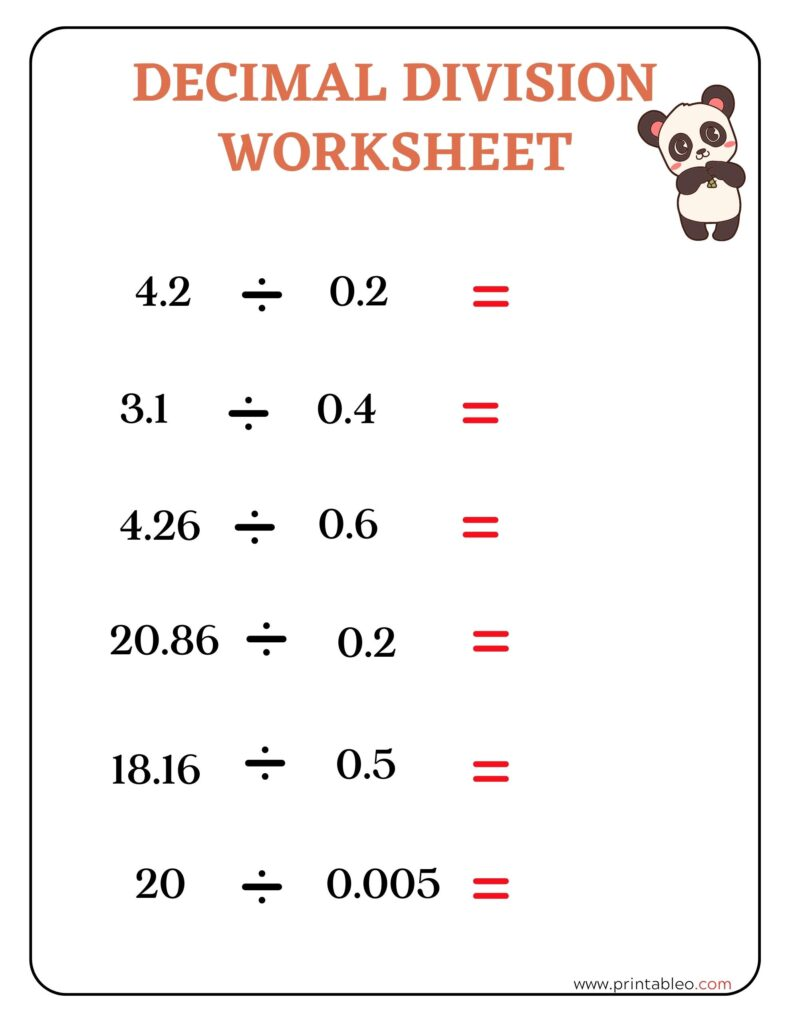## 10. Decimals Division Worksheets: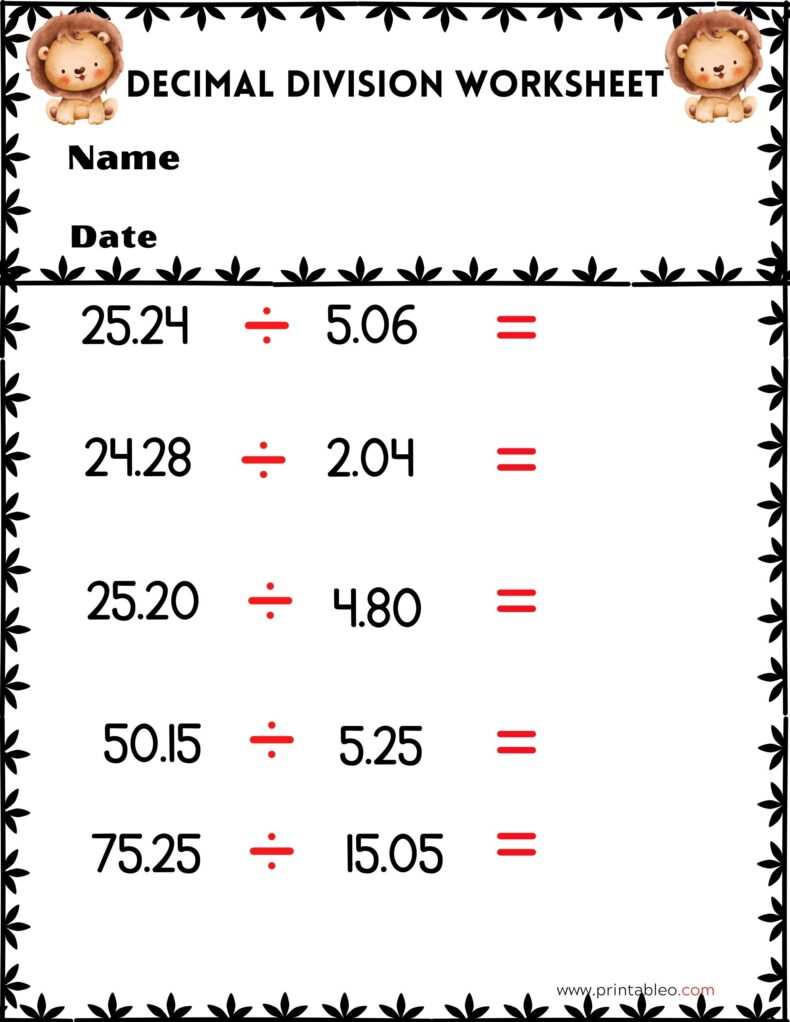## 11. Decimals Division Worksheets Third Grade:## 12. Decimals Division Worksheets Fifth Grade: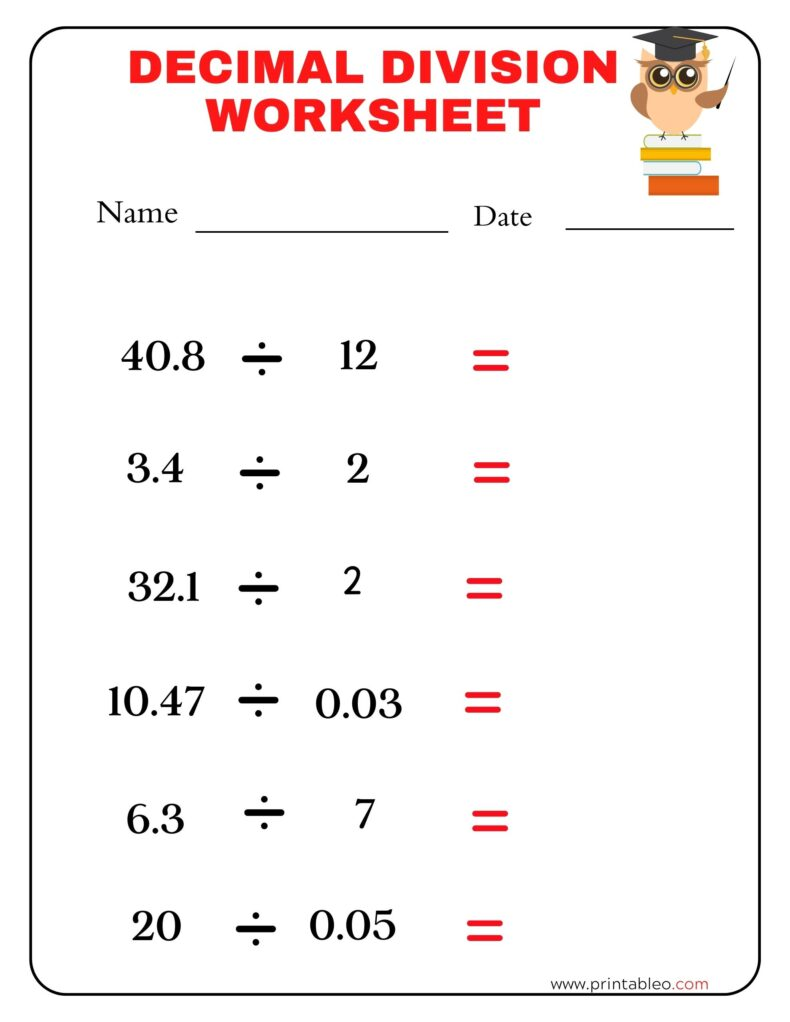## 13. Decimals Division Worksheets 5th Grade: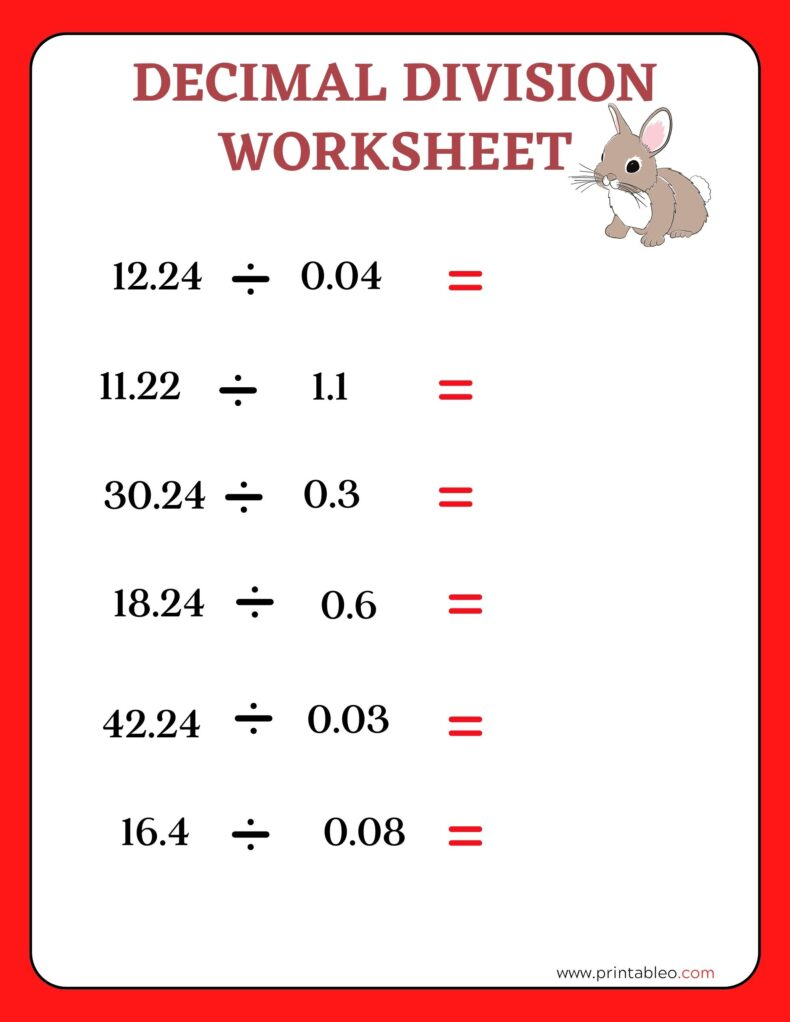## 14. Decimals Division Worksheets 4th Grade: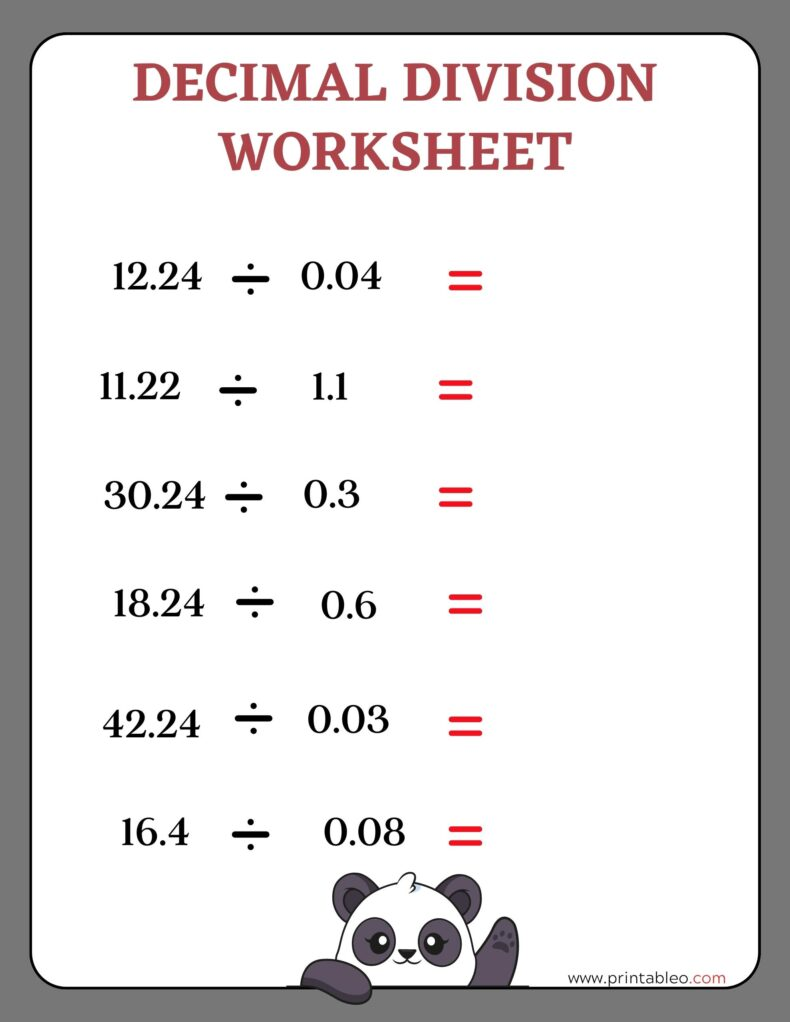## 15. Decimals Division Worksheets 3rd Grade: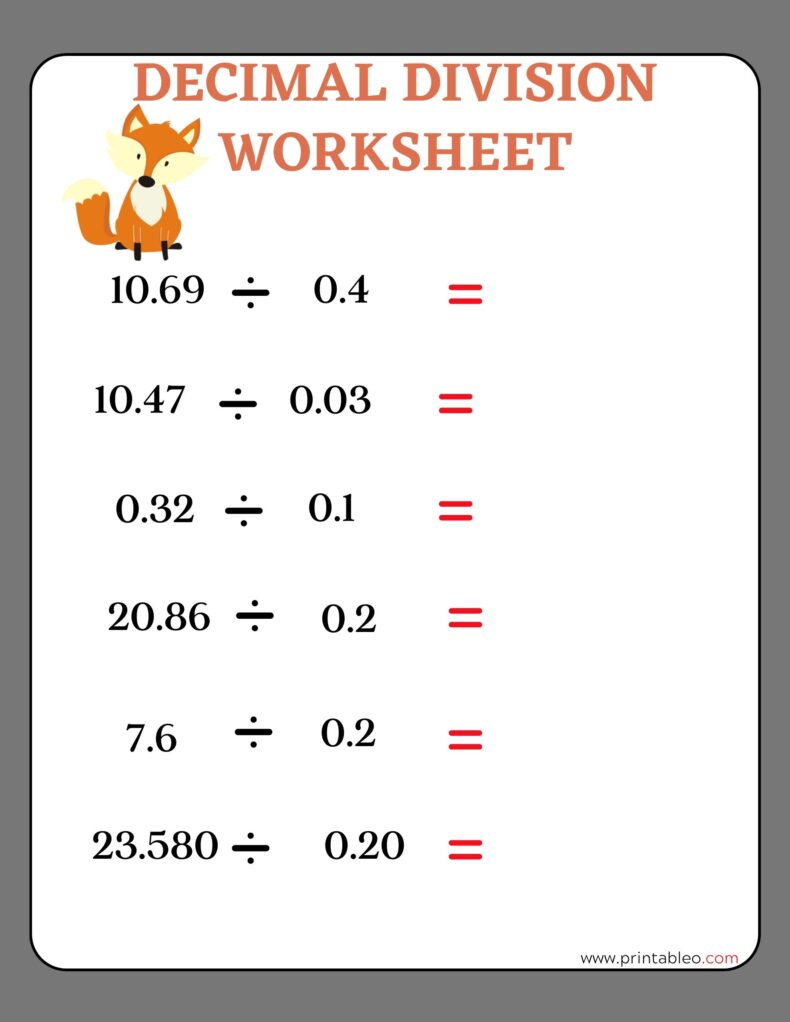## 16. Decimals Division Worksheet: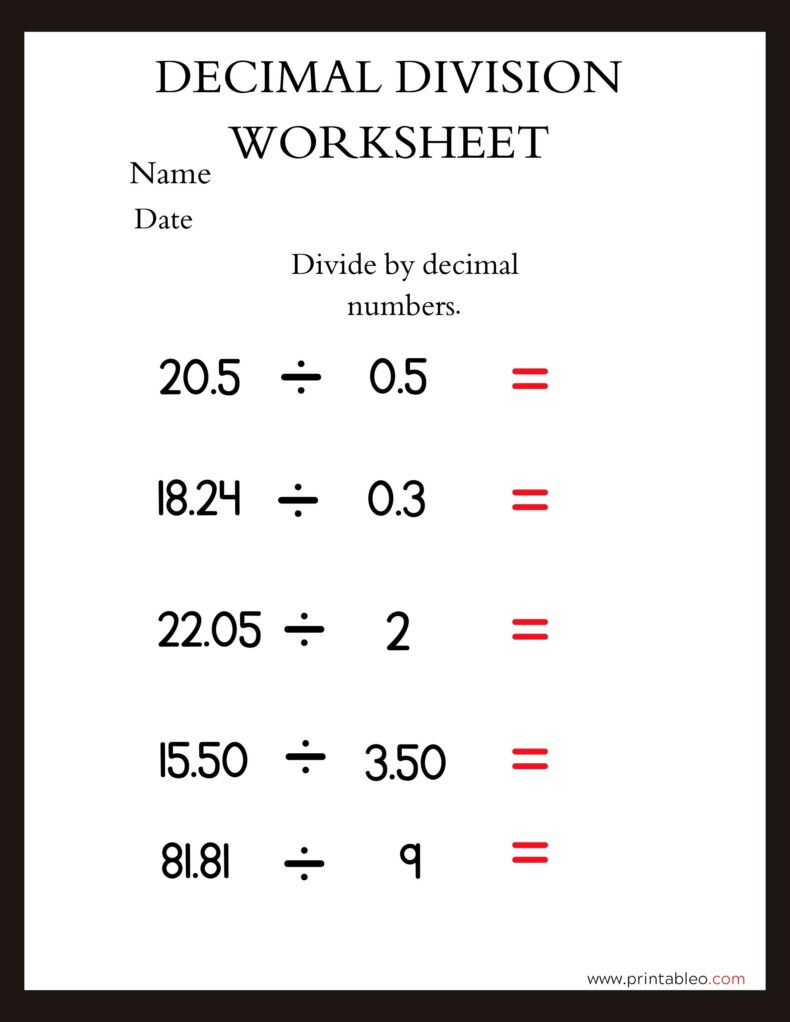## 17. Decimal Division Worksheets: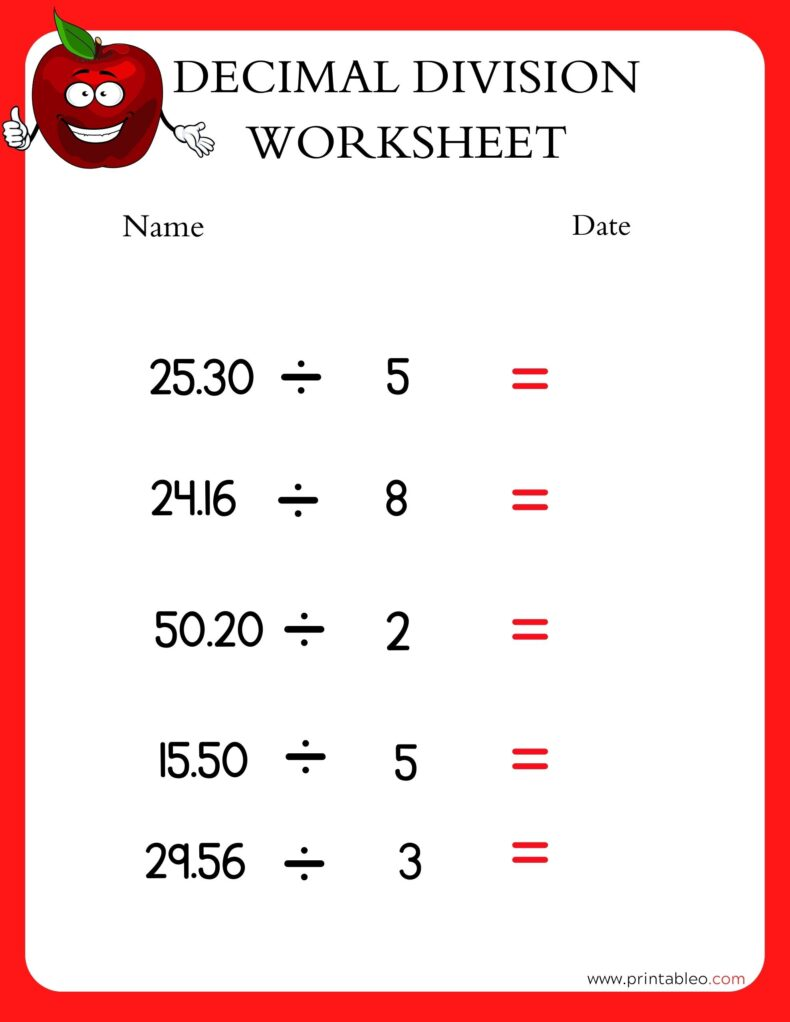## 18. Decimal Division Worksheet Third Grades: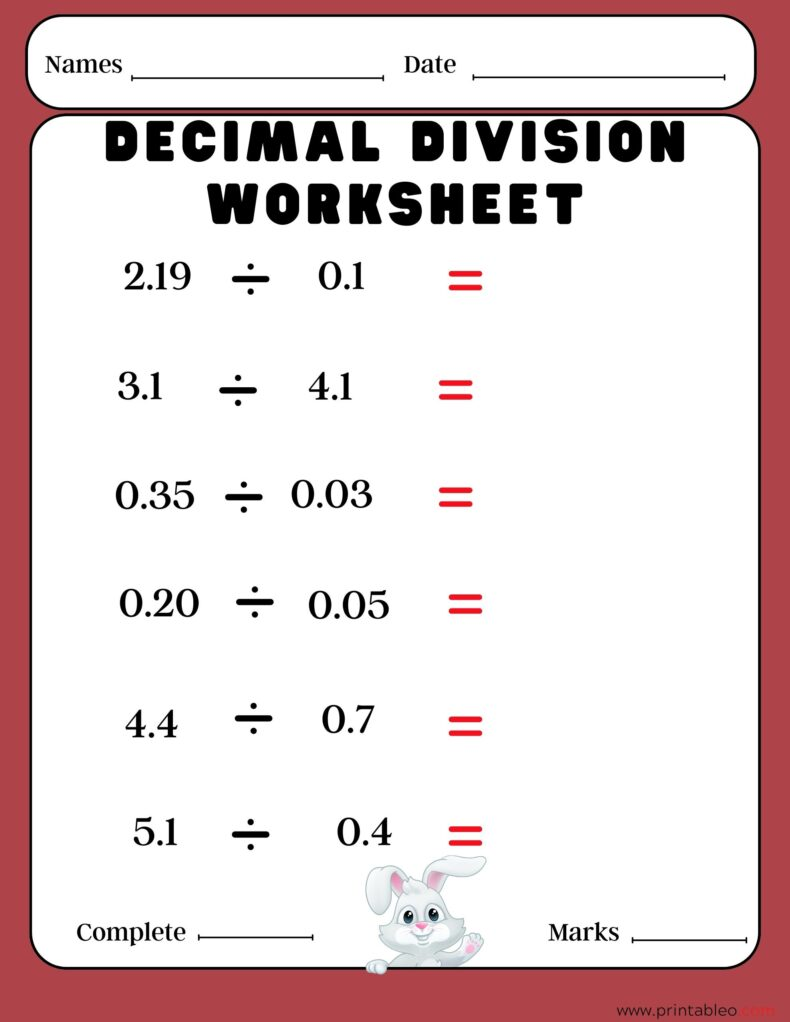## 19. Decimal Division Worksheet Sixth Grades: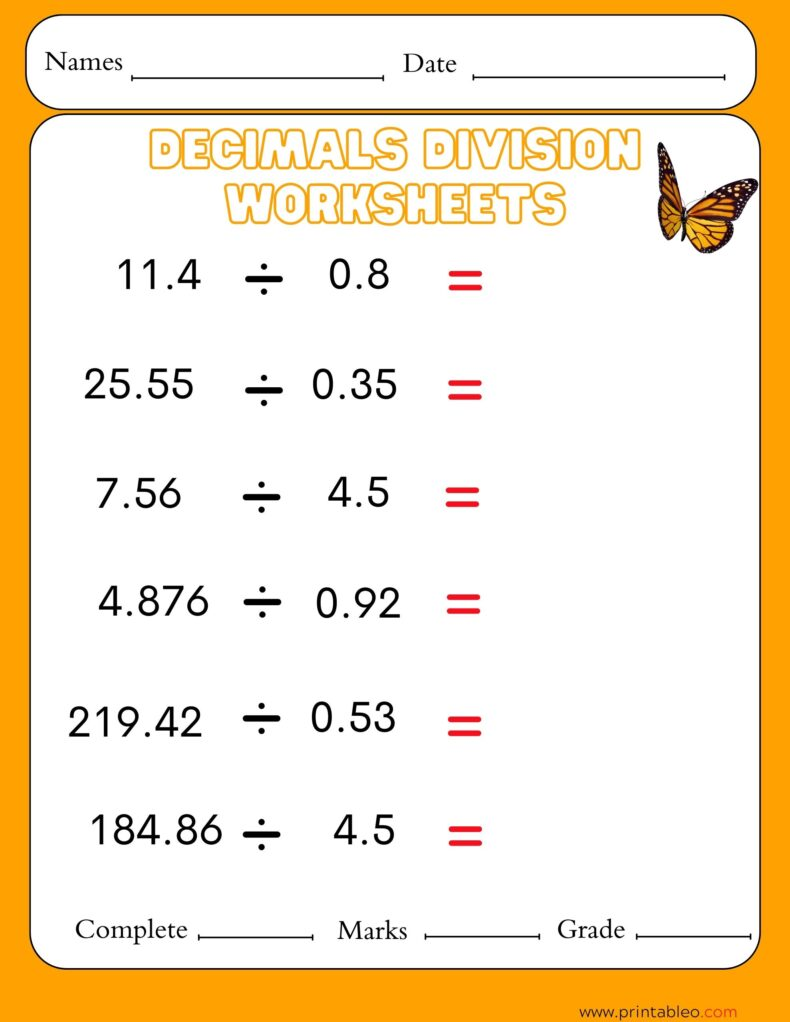## 20. Decimal Division Worksheet Forth Grades: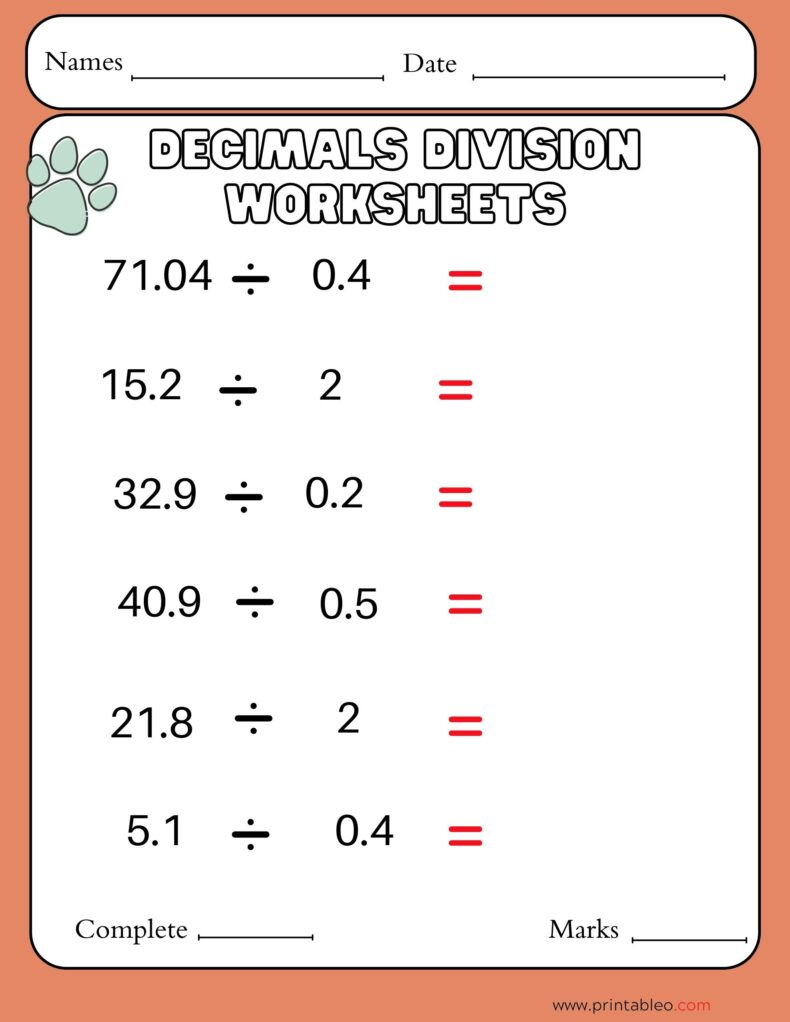## 21. Division Worksheets Decimals 4th Grade: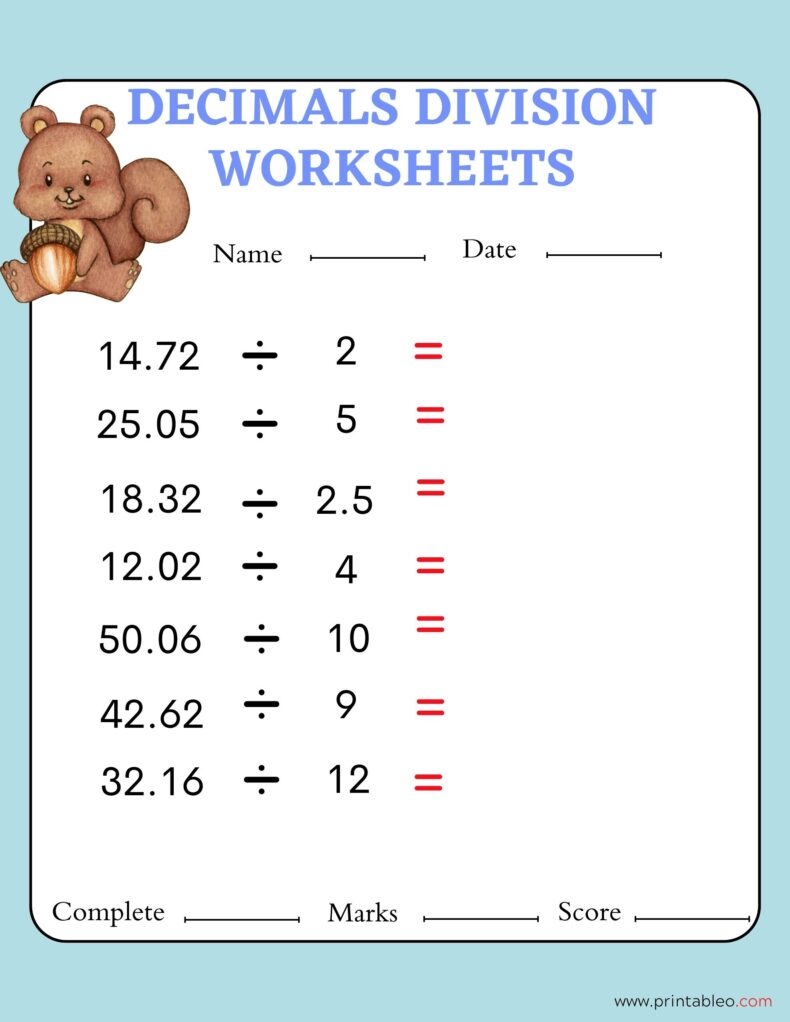## 22. Division Worksheets Decimals 3rd Grade: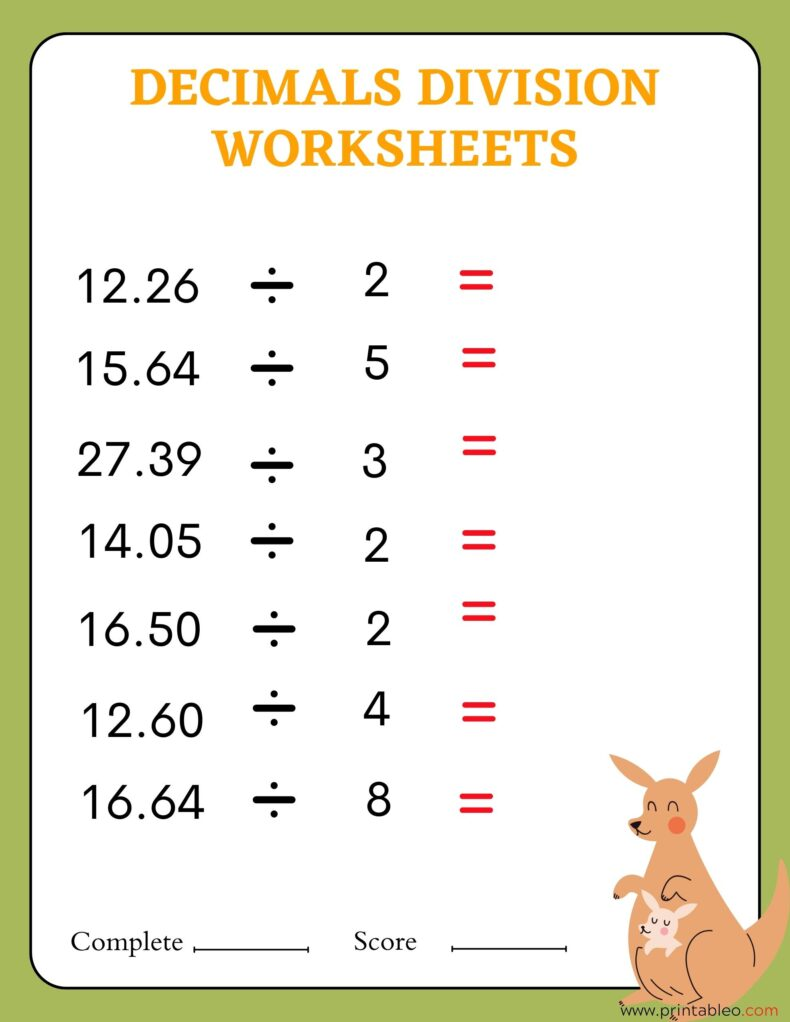## 23. Division With Decimal Worksheets: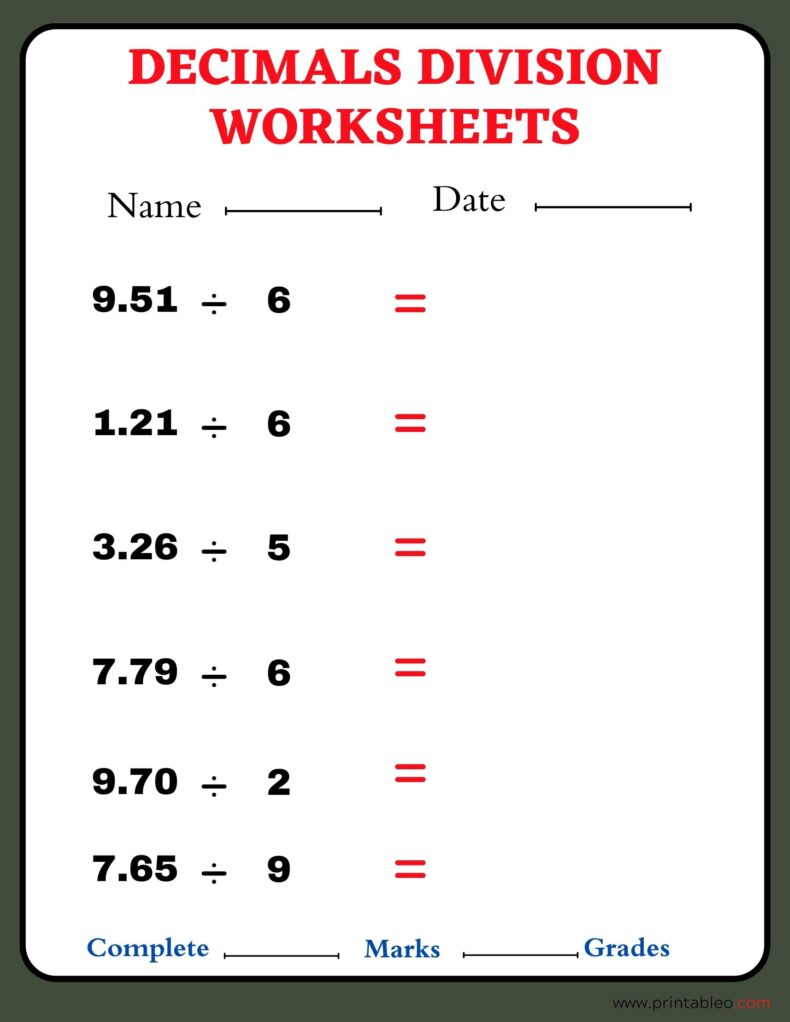## 24. Division With Decimal Worksheets Third Grade: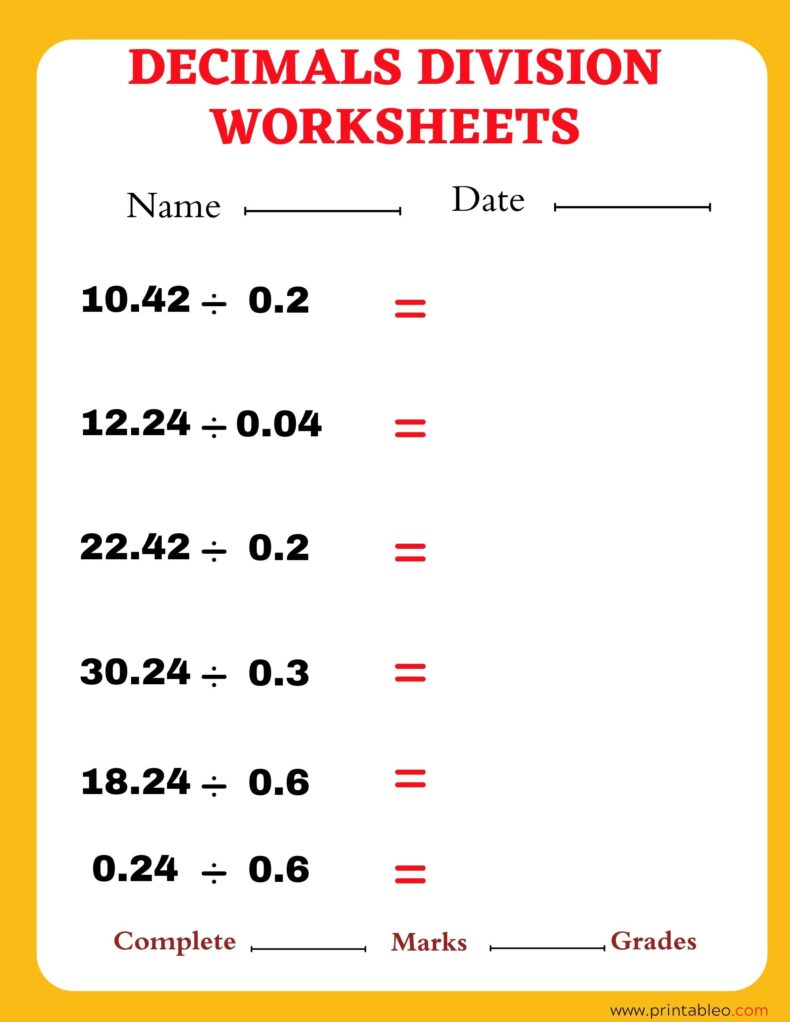## 25. Division With Decimal Worksheets Fourth Grade: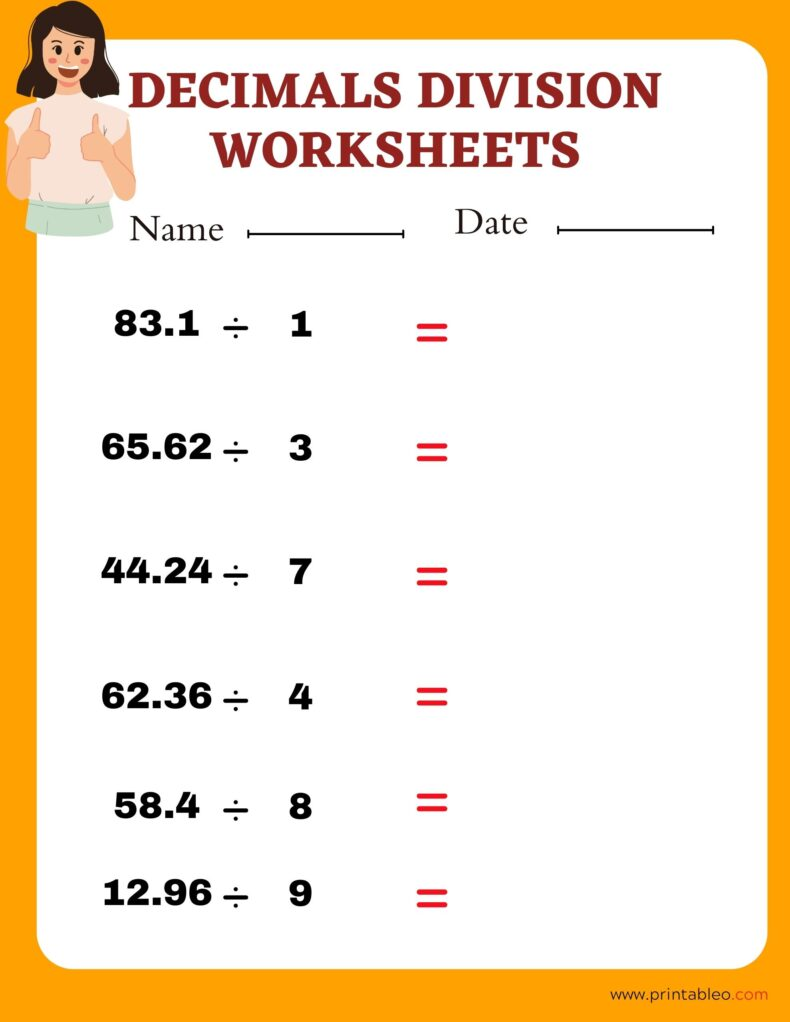## 26. Division With Decimal Worksheets Fifth Grade: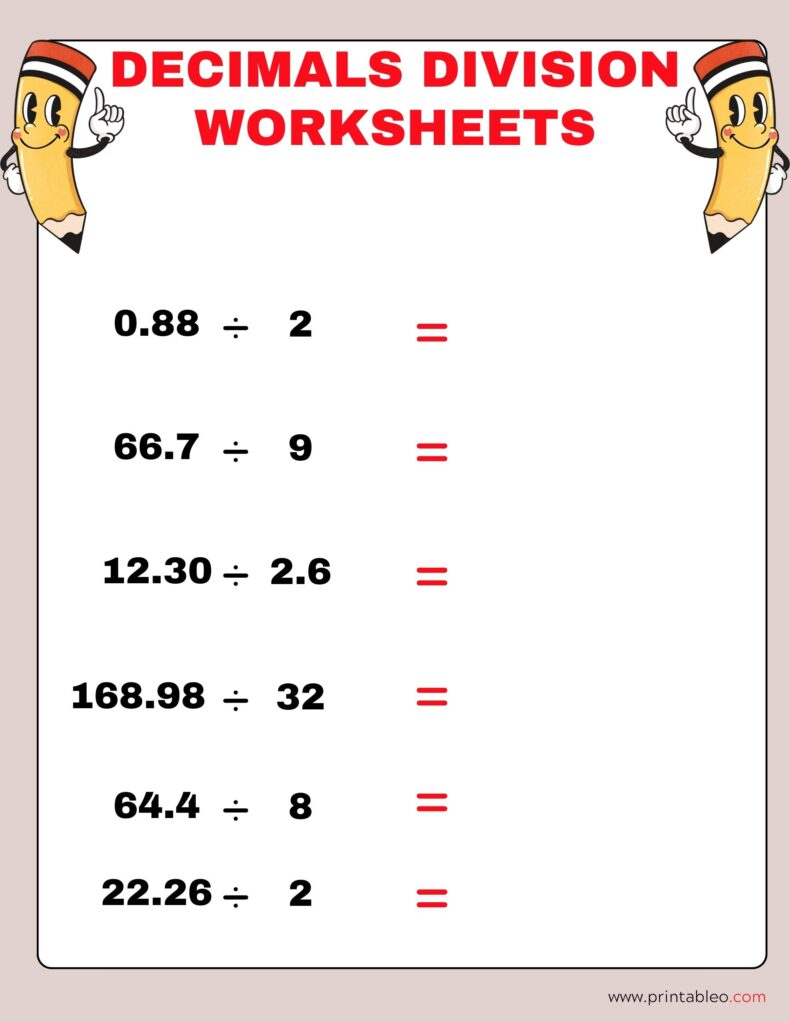## 27. Division of Decimals Worksheet: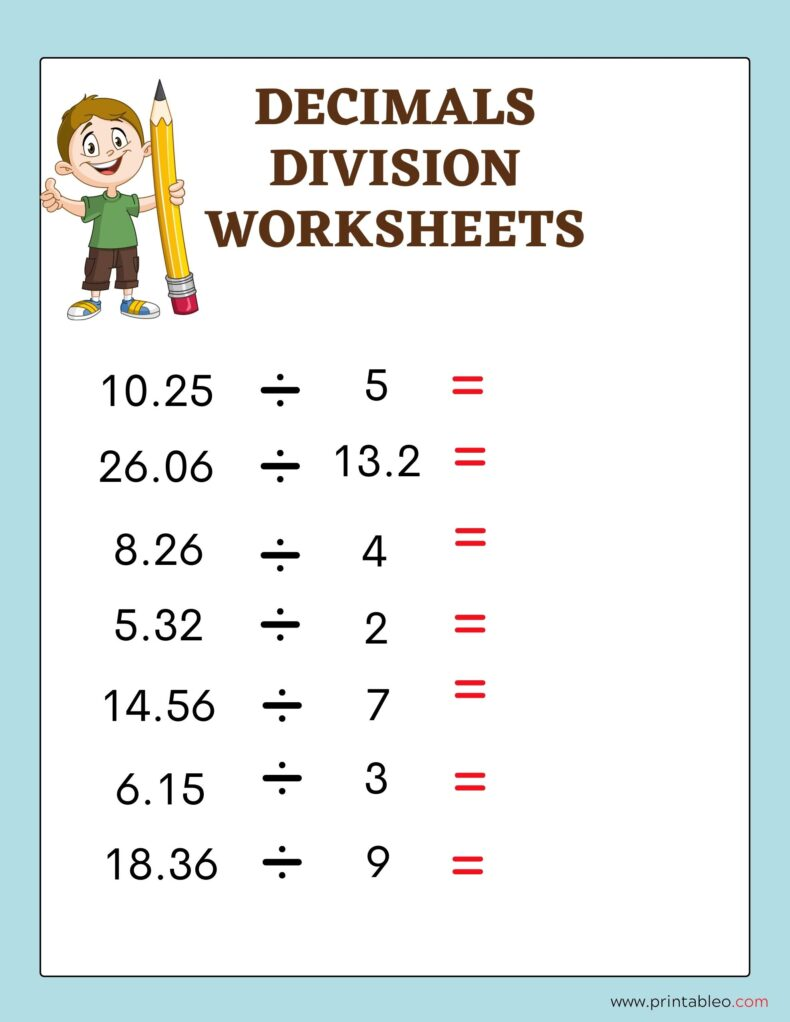## 28. Division of Decimals Worksheet Sixth Grade: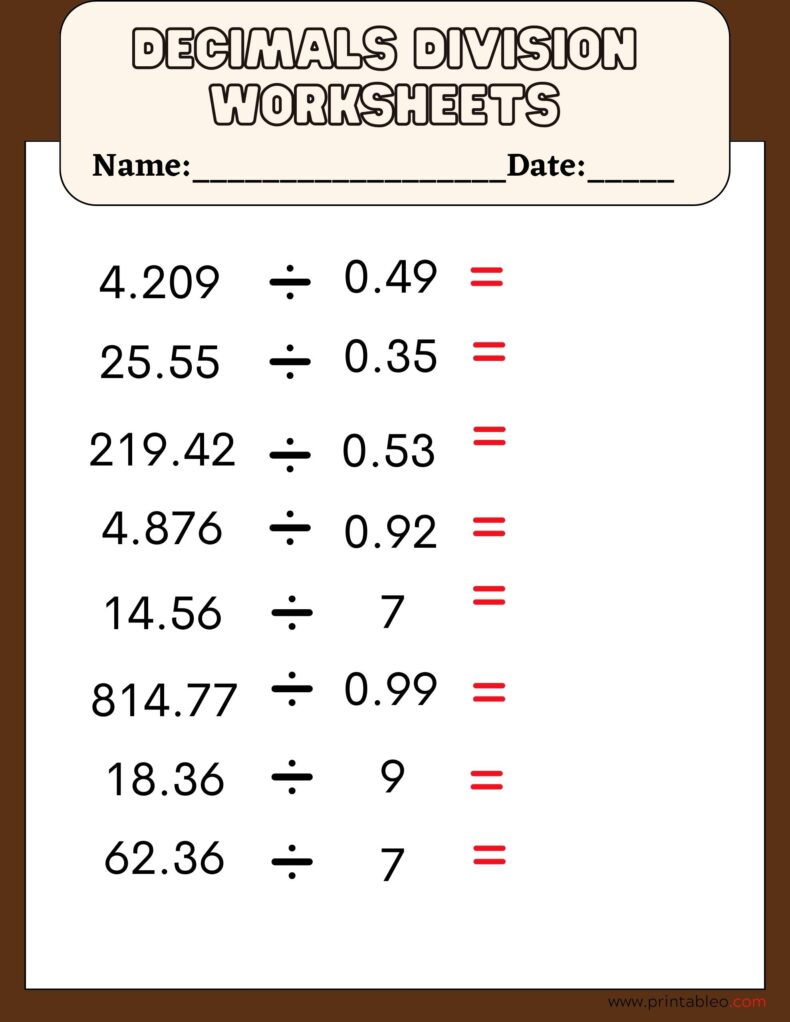## 29. Division of Decimals Worksheet Second Grade: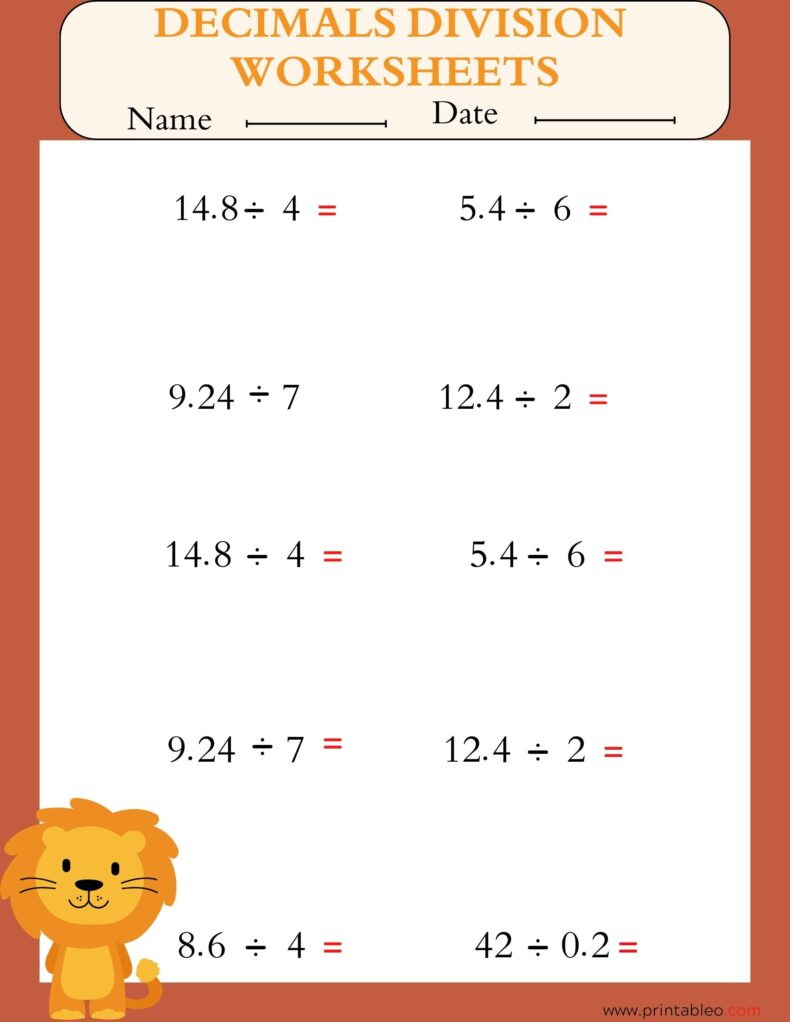## 30. Division of Decimals Worksheet First Grade: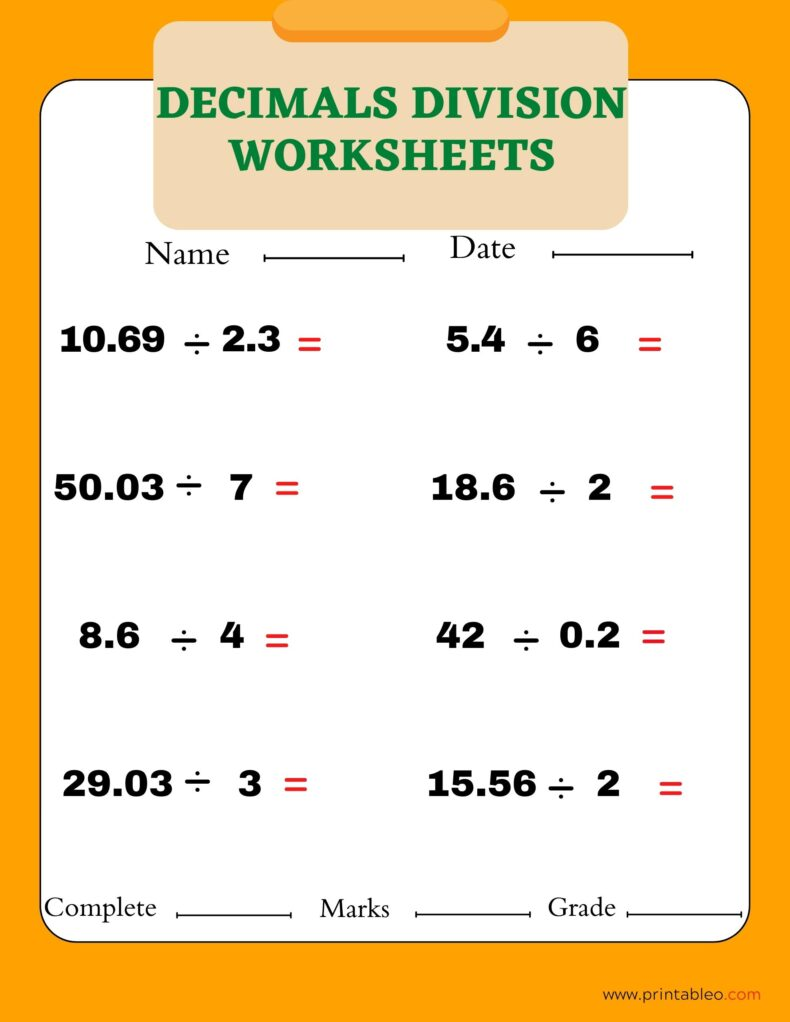## 31. Division of Decimals Worksheet Fifth Grade: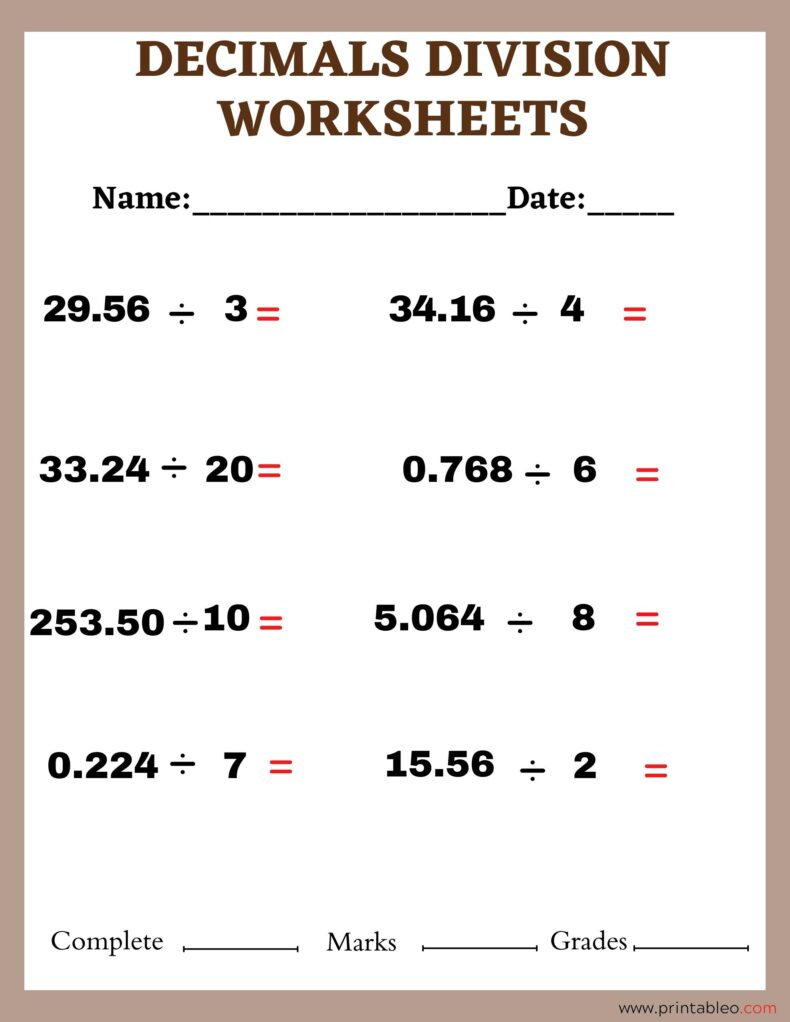## 32.Division of Decimals Worksheet 6th Grade: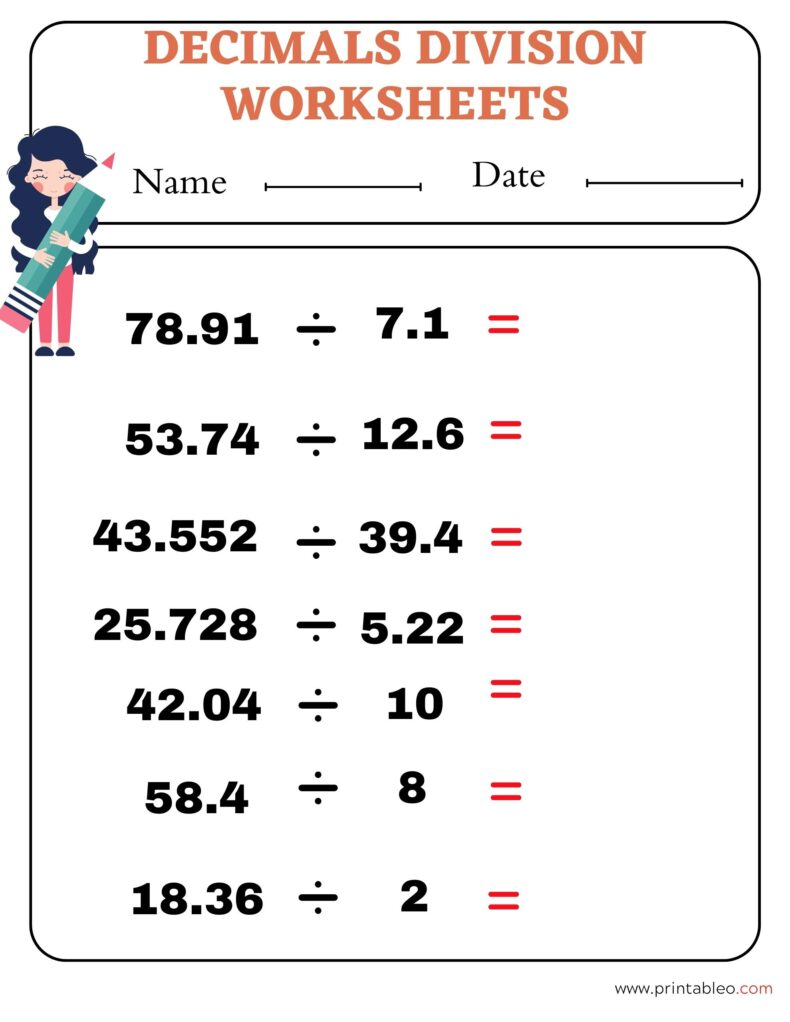## 33. Division of Decimals Worksheet 5th Grade: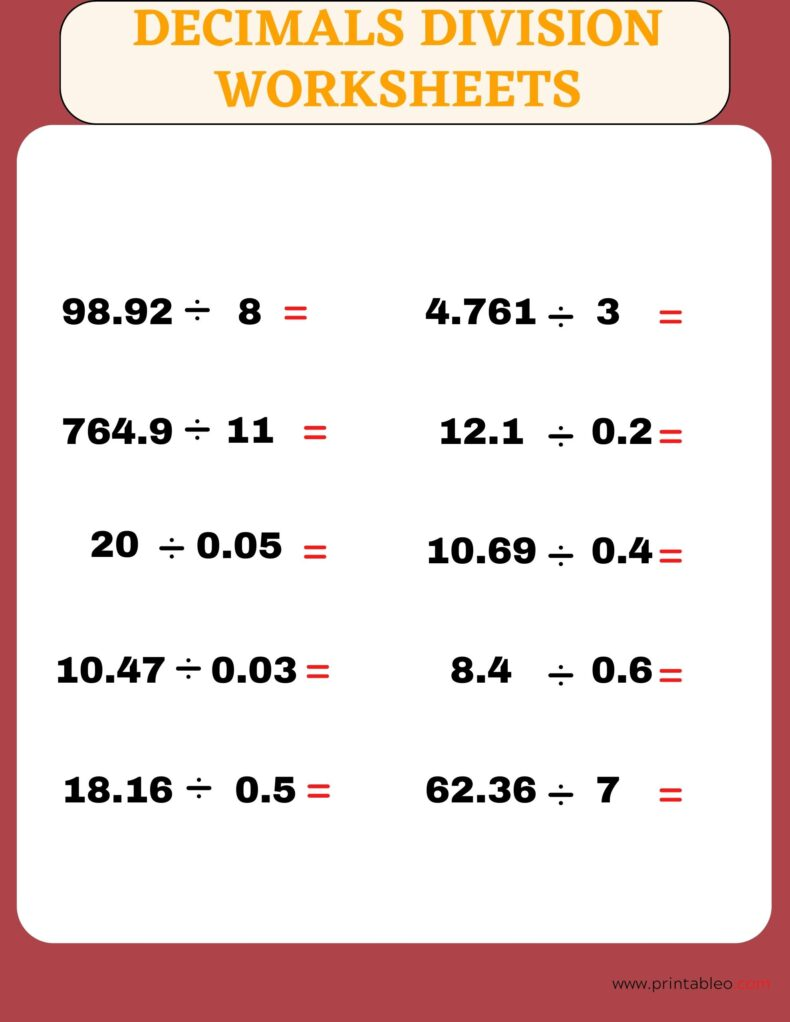## 34. Division of Decimals Worksheet 4th Grade: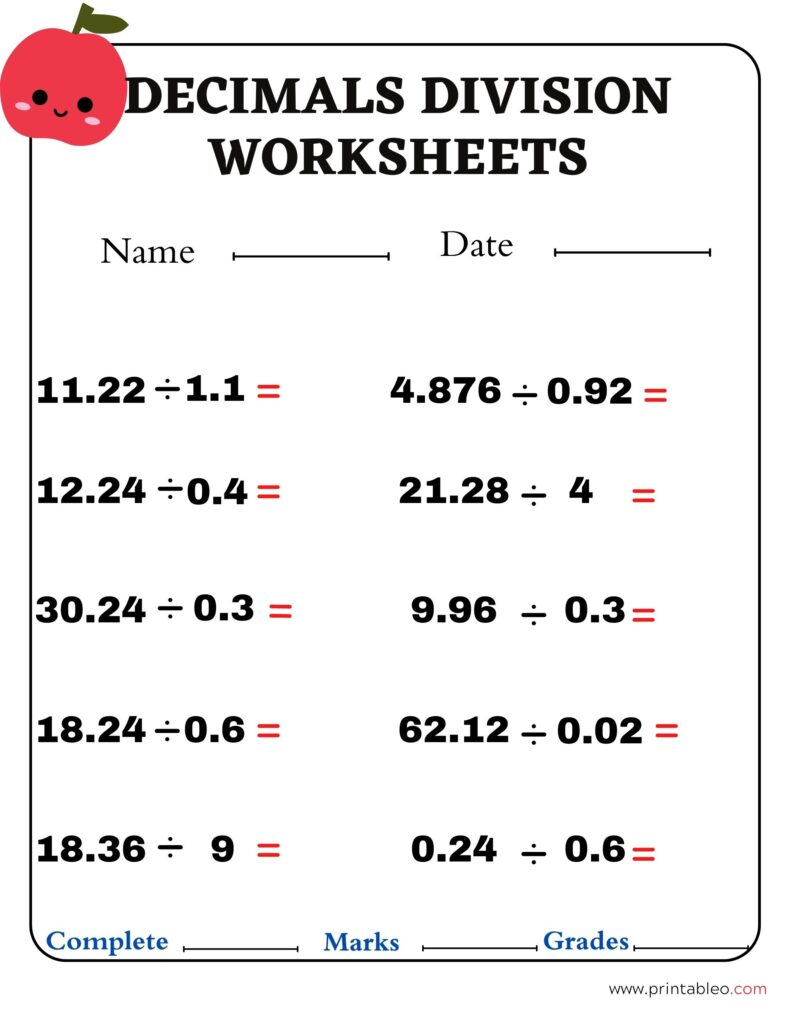## 35. Division Worksheets Decimals:## 36. Division Worksheets Decimals Third Grade: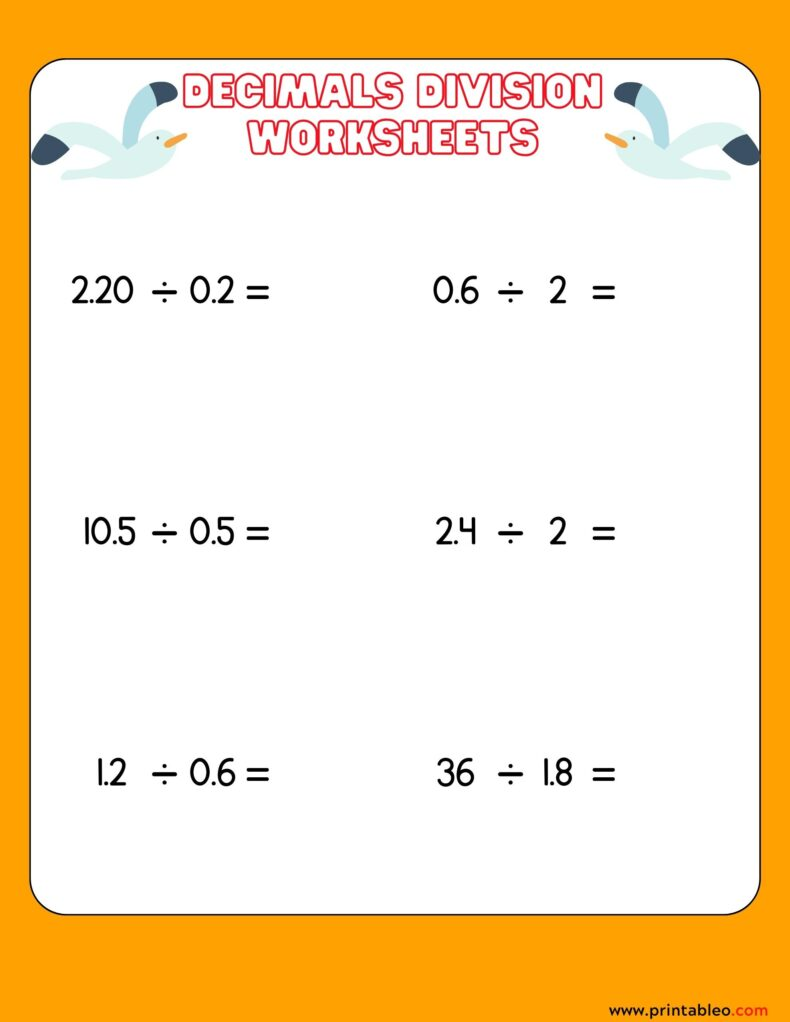## 37. Division Worksheets Decimals Sixth Grade: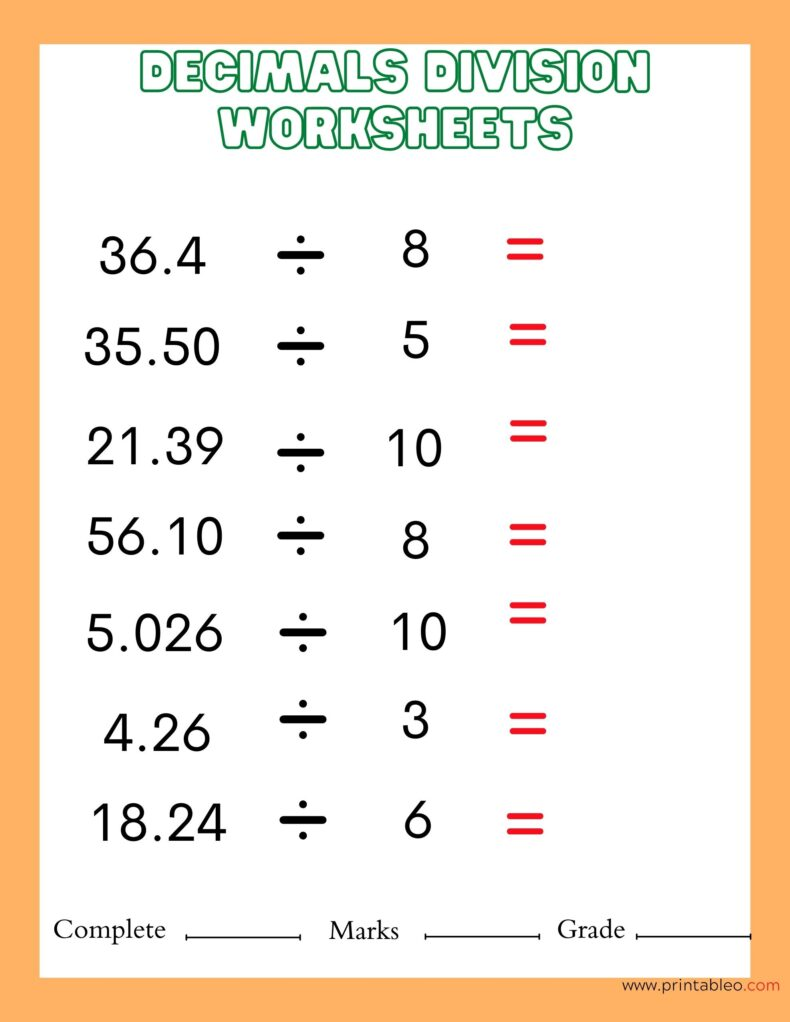## 38. Division Worksheets Decimals Fourth Grade: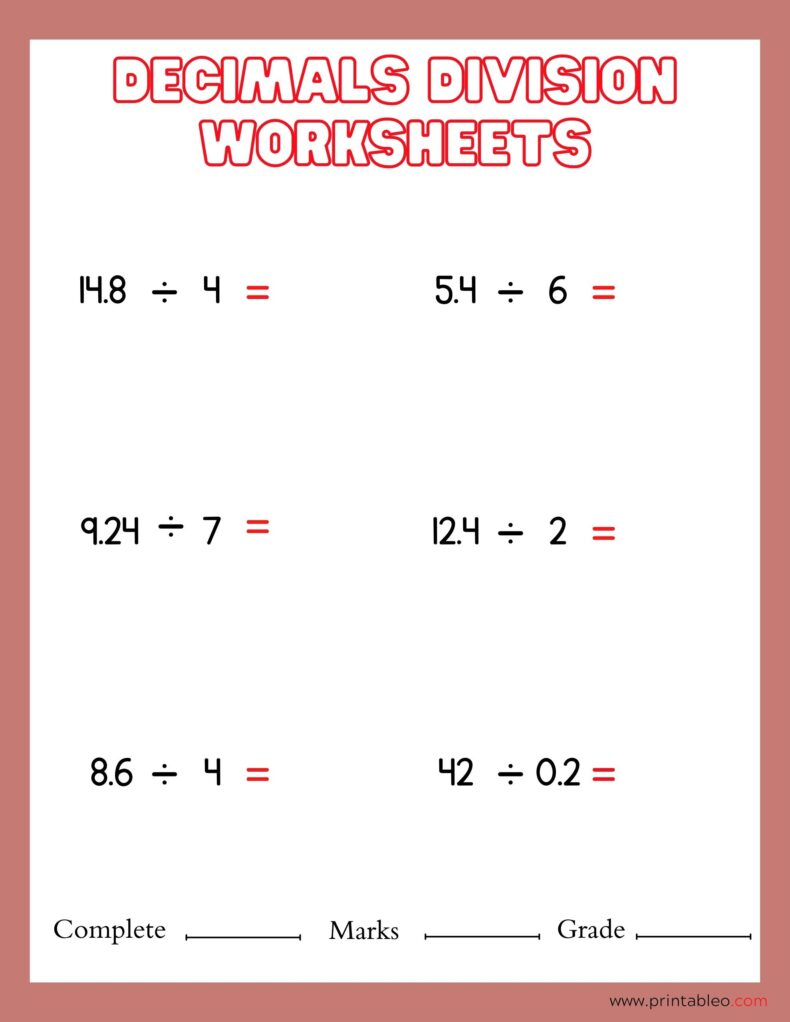## 39. Division Worksheets Decimals Fifth Grade: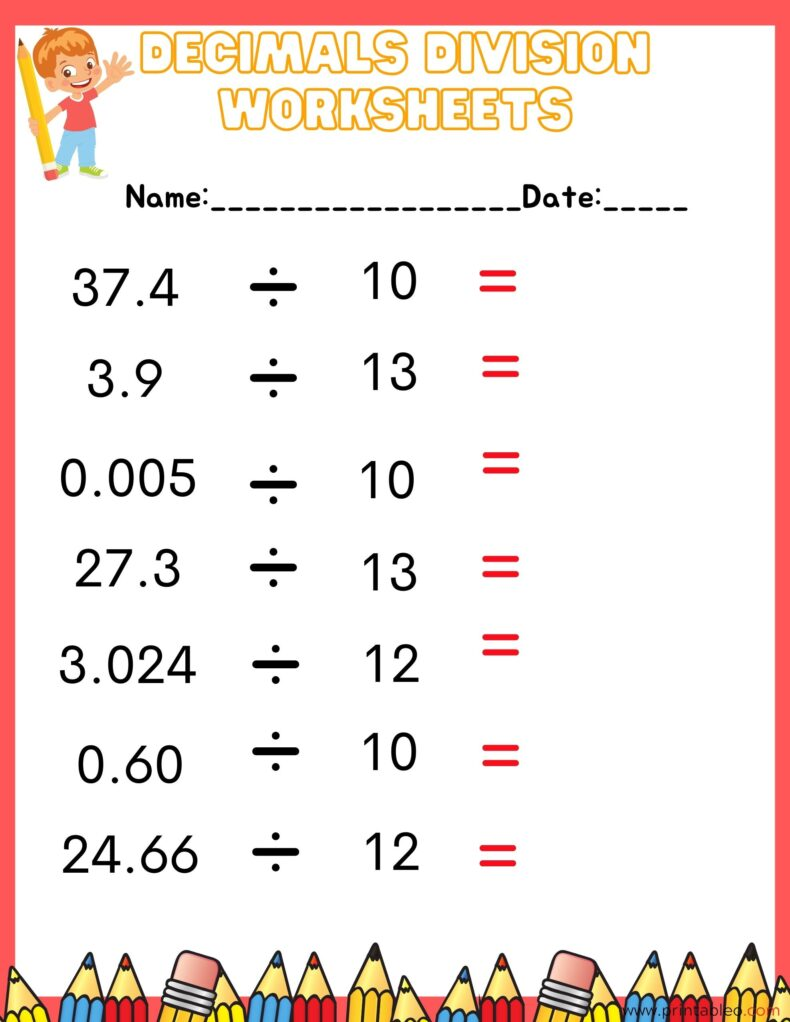## 40. Division Worksheets Decimals 6th Grade: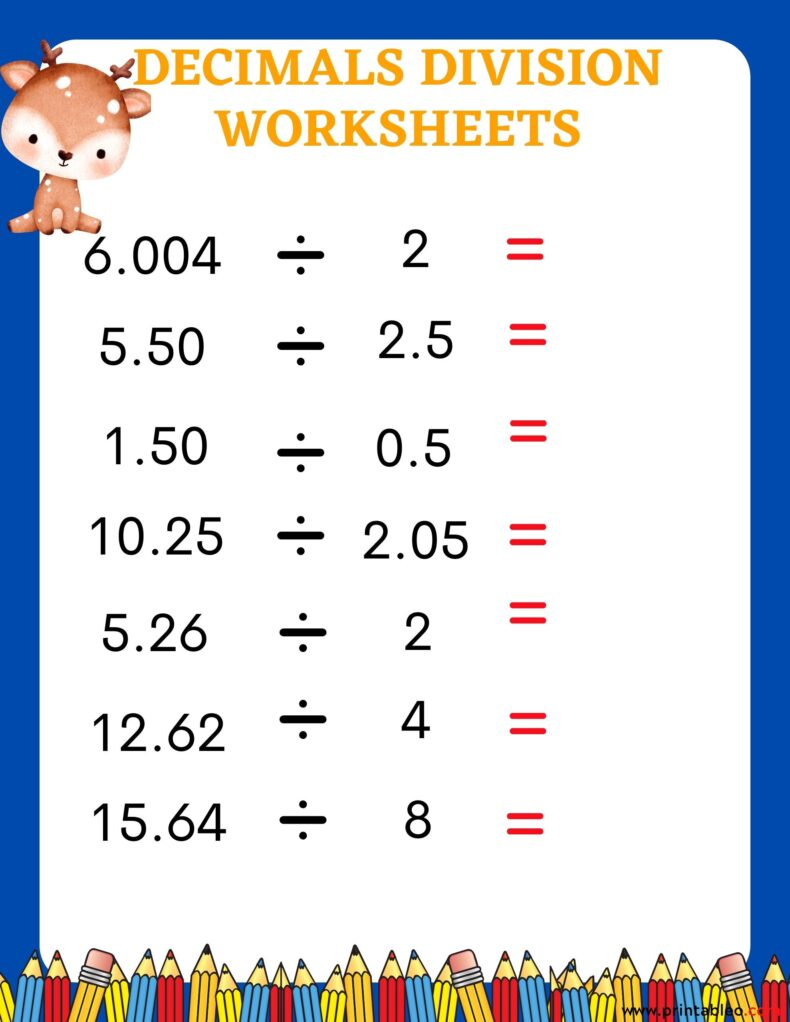## 41. Division Worksheets Decimals 5th Grade: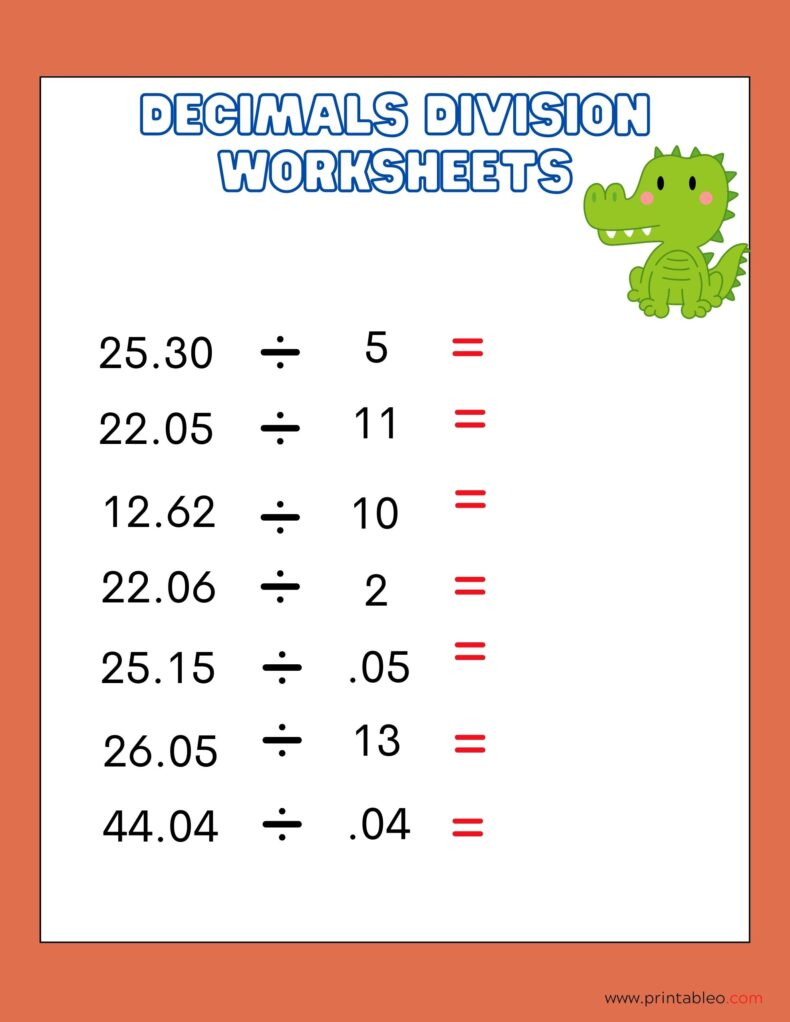### 15+Printable Pool Closed Sign